# Hales-Jewett theorem

(diff) ← Older revision | Latest revision (diff) | Newer revision → (diff)

Jewett–Hales theorem

One of the fundamental results in Ramsey theory.

Let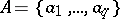be a finite alphabet and letdenote the set of-tuples with entries from. A set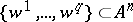consisting of-tuples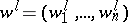,, is a combinatorial line if there exists a non-empty subset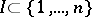such that forand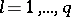one has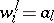and for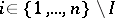one has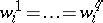. An alternative way of describing a combinatorial line is the following. Let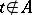be a "variable" . A variable word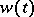is a word over the alphabet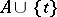in whichoccurs. For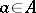, denote bythe word which results from replacing each occurrence ofinby. Then the set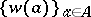is a combinatorial line. For example, ifand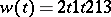then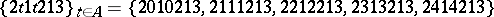is a combinatorial line in.

The Hales–Jewett theorem, proved in [a1], states that for any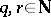there exists an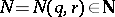such that if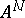is partitioned intoclasses, then at least one of these classes contains a combinatorial line.

The following is an equivalent formulation of the Hales–Jewett theorem. Given a set, let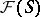denote the set of all finite subsets of. For anythere exists an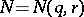so that ifis a set with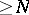elements, then for any-colouring of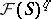there exist a non-empty set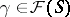and sets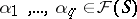such that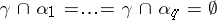and such that,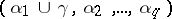,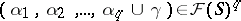all have the same colour.

Introduced originally in [a1] as a tool for analyzing certain types of games (cf. also Games, theory of), the Hales–Jewett theorem was soon recognized as a cornerstone of Ramsey theory. Among the numerous corollaries of the Hales–Jewett theorem one has the van der Waerden theorem on arithmetic progressions and its multi-dimensional version (the Gallai theorem). For example, to see that the Hales–Jewett theorem implies the van der Waerden theorem, take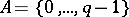and interpret the words overas base-expansions. Ifis a variable word over, thenis a length-arithmetic progression in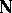. Also, in [a3] the Hales–Jewett theorem has been used to provide a significantly simplified proof of the geometric Ramsey theorem due to R. Graham, K. Leeb and B. Rothschild ([a2]). See [a4] for more details and discussion.

The Furstenberg–Katznelson density Hales–Jewett theorem ([a5]), which confirmed a conjecture made by Graham and which bears the same relation to the Hales–Jewett theorem as the Szemerédi theorem on arithmetic progressions bears to van der Waerden's theorem, states that for anyandthere exists anso that if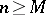,is a-element set andsatisfies, thencontains a combinatorial line.

A polynomial extension of the Hales–Jewett theorem was obtained in [a6], where it was shown that for any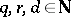there exists anso that ifis a set withelements, then for any-colouring ofthere exist a non-empty setand sets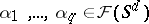such that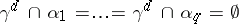and such that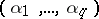,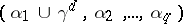,,,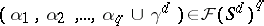all have the same colour. The method utilized in [a6] is that of topological dynamics; see [a7] or [a8] for a purely combinatorial proof. See [a9], [a10], [a11] for applications of the polynomial Hales–Jewett theorem to density Ramsey theory.

Infinitary generalizations of the Hales–Jewett theorem were obtained in [a12], [a13], [a14] and [a15]. For an infinitary version of the polynomial Hales–Jewett theorem, see [a7], Sect. 2.6.

For a proof of the Hales–Jewett theorem which yields a primitive recursive upper bound for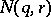, see [a16] or [a17].

It is conjectured that the polynomial Hales–Jewett theorem should admit a density generalization. See [a18], p. 57, for a formulation.

How to Cite This Entry:
Hales-Jewett theorem. Encyclopedia of Mathematics. URL: http://encyclopediaofmath.org/index.php?title=Hales-Jewett_theorem&oldid=16392
This article was adapted from an original article by V. Bergelson (originator), which appeared in Encyclopedia of Mathematics - ISBN 1402006098. See original article